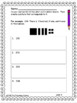# Math WritingSubject
Resource Type
File Type

PDF

(15 MB|164 pages)
Standards
• Product Description
• Standards

This bundle has page after page of math writing. Just print and go. There are 3 levels for each standard that is in this packet. The math writing is aligned to common core standards for 2nd. The purpose of math writing is to reinforce math skills and develop an understanding of math concepts. Math writing gives the teacher an idea of what the students understands, does not understand, and gives the teacher an idea of how the student is thinking.

Each page is marked at the bottom of the page as Level 1, Level 2, or Level 3.

Included in this packet:

Math Graphic Organizers

Greater than, Less than

Greater than, Less than True or False

Great than, Less than Word Problems

Even/Odd

Place Value

Letter writing--subtraction without regrouping

Letter writing--subtraction with regrouping

Word Problem

Subtraction using base ten (picture)

Skip Counting

Identifying Money

Spending Money

Geometry

Identifying Geometry

Graphs with shapes

Measurement

Multiplication (creating word problems)

Multiplication (Letter writing)

Arrays

Number Lines

Time

Write opinion pieces in which they introduce the topic or book they are writing about, state an opinion, supply reasons that support the opinion, use linking words (e.g., because, and, also) to connect opinion and reasons, and provide a concluding statement or section.
Recognize and draw shapes having specified attributes, such as a given number of angles or a given number of equal faces. Identify triangles, quadrilaterals, pentagons, hexagons, and cubes.
Determine whether a group of objects (up to 20) has an odd or even number of members, e.g., by pairing objects or counting them by 2s; write an equation to express an even number as a sum of two equal addends.
Explain why addition and subtraction strategies work, using place value and the properties of operations.
Add and subtract within 1000, using concrete models or drawings and strategies based on place value, properties of operations, and/or the relationship between addition and subtraction; relate the strategy to a written method. Understand that in adding or subtracting three-digit numbers, one adds or subtracts hundreds and hundreds, tens and tens, ones and ones; and sometimes it is necessary to compose or decompose tens or hundreds.
Total Pages
164 pages
N/A
Teaching Duration
30 minutes
Report this Resource to TpT
Reported resources will be reviewed by our team. Report this resource to let us know if this resource violates TpT’s content guidelines.